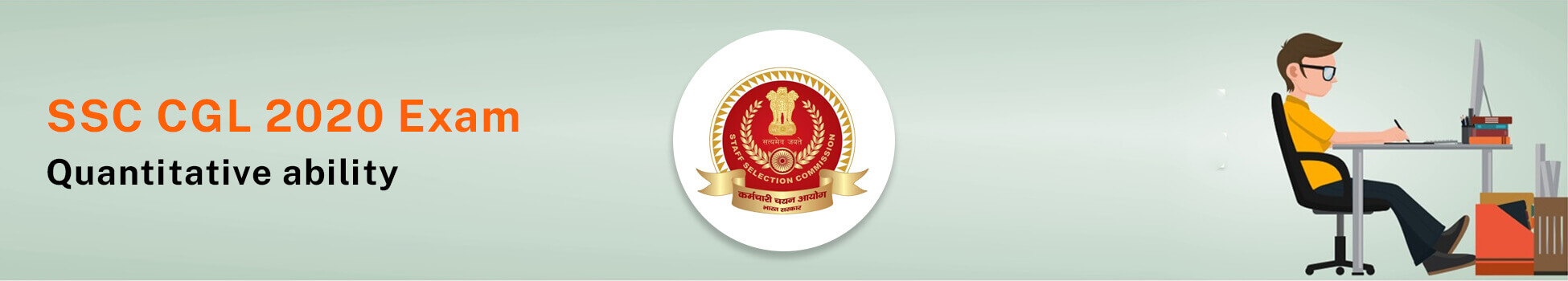SSC CGL 2020: Quantitative Aptitude - Study24x7Default error msg

New to Study24x7 ?

# SSC CGL 2020: Quantitative Aptitude

Updated on 03 March 2020SSC Preparation Strategies & S
Updated on 03 March 2020SSC CGL new vacancy 2020 has been out.

One of the most scoring topics for SSC CGL 2020 is quantitative aptitude.

Mock test in Hindi can also help you to practice more and score well.

SSC CGL 2020:  Percentage

Percentage (%) is a fraction or ratio where denominator is 100.

1. n% of x means

( x * n)/100

Example: 20% of 150 is (20*150)/100 =30

1. n% of x= x% of n

Example: 20% of 5= 5% of 20

(5*20)/100= (20*5)/100=1

1. find what percentage of x is n

(n / x)*100

1. If x is increased/decreased by n, then percentage

x (1+ n/100)1. Percentage change in value= (change/initial value)*100
2. If  price of a commodity increased/decreased by N%, then change in consumption without changing the expenditure will be:

(N/ (100 + N)) * 100 %1. If the Population (P) is increasing with  rate R% annually then,

Population after n years = P*(1 + R/100) n

Population before n years = P/ (1 + R/100) n

1. If  price (P) of a commodity depreciates R% annually then,

Price of commodity after n years = P*(1 - R/100) n

Price of commodity n years ago = P/ (1 - R/100) n

SSC CGL 2020: Ratio and Proportion

Ratio(x/y) is the relation between two or more values of same kind.

Where x is antecedent and y is consequent.

For example: x: y is not same as y: x.

Proportion (::) is when ratio of first and second element is equal to third and fourth element.

A: B = C: D

For example: 4:10:: 8: 20=2/5

Fourth Proportional (D) = (B*C)/ A

Third proportional (C) = B2/A

SSC CGL 2020: Speed, Distance & Time

Measurements:

Speed in km/hr or m/sec or miles/ hr.

Distance in Km or meter or miles.

Time is in hours or minutes or seconds.

Conversions:

Km/hr to meter/ sec = speed in km/hr* 5/18

Meter/sec to km/hr= speed in m/sec* 18/5

Formulas:

1.  Distance= Speed X Time

1. Speed=Distance/Time

1. Time= Distance/ Speed

1. If  object is moving with x and y speed, then

Average speed = (2xy)/(x + y)

1. When two objects are moving in same direction with x and y speed then

Relative speed = difference of x and y

1. When two objects are moving in different direction  with x and y. then,

Relative speed = addition of x and y

1. If time taken by objects is same, then

Average speed = (x + y)/2

1. If distance covered by objects is same, then

Average speed = 2x*y/ (x + y)

1. If two people moving between two points A and Bin opposite direction by crossing each other, then speed of them will be:Speed of A/ Speed of B=√Time taken by B/ Time taken by A

1. Calculation of least time taken to meet in circular race is LCM (T1, T2, T3…… Tn) i.e. Least common Multiple.

Write a comment...Trending ArticlesBy CBSE CLASS 8By Edubabble LLPBy CBSE CLASS 7By CBSE CLASS 10
Related PostsServicesNeed Some Help!Connect